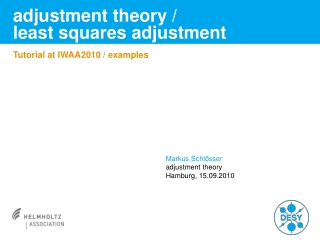DownloadDownload PresentationTélécharger la présentation- - - - - - - - - - - - - - - - - - - - - - - - - - - E N D - - - - - - - - - - - - - - - - - - - - - - - - - - -
##### Presentation Transcript

1. adjustment theory /least squares adjustment Tutorial at IWAA2010 / examples Markus Schlösser adjustment theory Hamburg, 15.09.2010

2. random numbers • Computer generated random numbers • are only pseudo-random numbers • Mostly only uniformly distributed prn are availiable (C, Pascal, Excel, …) • Some packages (octave, matlab, etc.) have normally distributed prn („randn“) • Normally distributed prn can be obtained by • Box-Muller method • Sum of 12 U(0,1) (is an example for central limit theorem) • ….

3. random numbers / distributions

4. random numbers / distributions

5. random variables / repeated measurements Random variable Observations „real“ value (normally unknown) normal distributed errors

6. random variables / repeated measurements Random variable Observations „real“ value (normally unknown) normal distributed errors

7. random variables / repeated measurements Random variable Observations „real“ value (normally unknown) normal distributed errors

8. random variables / repeated measurements Random variable blunder Observations „real“ value (normally unknown) normal distributed errors

9. error propagation • assume we have • instrument stand S • fixed point F • S and F both with known (error free) coordinates • horizontal angle to F and P, distance from S to P • instrument accuracy well known from other experiments • looking for • coordinates of P • confidence ellipse of P

10. error propagation Parameters Observations Point X [m] Y [m] rSF [gon] 321.6427 gon S 10.332 10.642 X = rSP [gon] = 14.9684 gon F 20.673 2.145 dSP [m] 10.2486 m P tSF 356.2119 gon standard deviation of observations Unknowns Z = XP [m] = 17.631 m Variance / Covariance Matrix YP [m] 17.836 m

11. error propagation F contains partitial derivative of j build the difference quotient (numerically) with 0.113004 -0.113004 0.712224 F = -0.114658 0.114658 0.701952

12. error propagation covariance matrix of unknowns SZZ = 0.022589 0.017666 0.017666 0.022076 variances of coordinates are on the main diagonal BUT, this information is incomplete and could even be misleading, better use Helmert‘s error ellipse:

13. error propagation or even better, use a confidence ellipse. That means that with a chosen probablity P the target point is inside this confidence ellipse. P = 0.99 (=99%) Quantil of -distribution, with 2 degrees of freedom A0.99 = 0.61mm B0.99 = 0.21mm Q = 50gon

14. network adjustment Example: Adjustment of a 2D-network with angular and distance measurements

15. adjustment theory • f = 0 • no adjustment, but error propagation possible • no control of measurement • f > 0 • adjustment possible • measurement is controlled by itself • f > 100 typical for large networks • f < 0 • scratch your head

16. network adjustment Name X [m] Y [m] • small + regular network • 2D for easier solution and smaller matrices • 3 instrument stands (S1, S2, S3) • 8 target points (N1 … N8) • all points are unknown (no fixed points) • initial coordinates are arbitrary, they just have to represent the geometry of the network N1 0.000 0.000 N2 10.000 0.000 N3 0.000 10.000 N4 10.000 10.000 N5 0.000 20.000 N6 10.000 20.000 N7 0.000 30.000 N8 10.000 30.000 S1 5.000 5.000 S2 5.000 15.000 S3 5.000 25.000

17. network adjustment - input vector ofcoarse coordinates vector of observations vector ofstandard deviations vector ofunknowns

19. network adjustment – design matrix

20. network adjustment A-Matrix has lots of zero-elements orientation unknowns instrument stands Network points

21. network adjustment P is a diagonal matrix, because we assume that observations are uncorrelated

22. network adjustment • Normal matrix shows dependencies between elements • Normal matrix is singular when adjusting networks without fixed points • easy inversion of N is not possible • network datum has to be defined • add rows and colums, to make the matrix regular

23. network adjustment • datum deficiency for 2D-network with distances: • 2 translations • 1 rotation • minimize the total matrix trace means to put the network on all point coordinates • additional rows and columns look as Constraints: No shift of network in x No shift of network in y No rotation of network around z

24. network adjustment • after addition of G, Normalmatrix is regular and thus invertible • N-1 is in general fully occupied

28. network adjustment building the covariance matrix of unknowns (with empirical s02) error probability 1-a 2D-Network degrees of freedom

29. network adjustment error ellipses with P=0.01 error probability for all network points

30. network adjustment confidence ellipses for all network points relative confidence ellipses beewen some network points

31. network adjustment Relative confidence ellipses are most useful in accelerator sience, because most of the time you are only interested in relative accuracy between components. For relative ellipse between N2 and N4 Ellipse parameters are then calculated from SrelN2N4

32. network adjustment estimation of s02 from corrections v is used as a statistical test, to proof that the model parameters are right à priori variances are ok, with P = 0.99

33. adjustment Example: 2D - ellipsoid fid deviation of position and rotation of an ellipsoidal flange

34. flange adjustment Observations known parameters (e.g. from workshop drawing) unknowns with initial value constraints

35. flange adjustment Since it is not (easily) possible to separate unknowns and observations in the constraints, we use the general adjustment model: B contains the derivative of j with respect to L A contains the derivative of j with respect to X k are the Lagranges Multiplicators (“Korrelaten”) x is the vector of unknowns w is the vector j(L,X0)